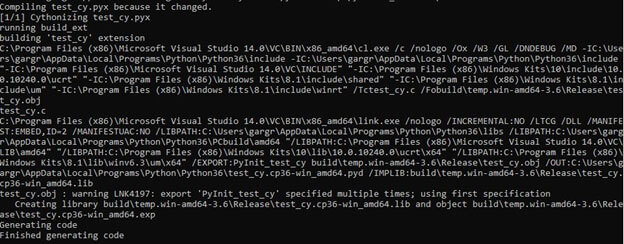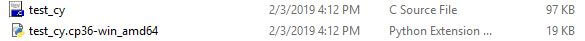﻿ How to build Python apps rocket boost using Cython?

How to make Python faster using Cython?

In this tutorial, we will discuss how to make Python run faster using Cython. First will take the general idea of Cython i.e., How it is working? How to install Cython? Further, we will take a look through example how to take advantage of it by little modifications in your code. Let’s get started to deep dive into the details.

Why do we need Cython?

Cython basically acts as an intermediary between Python and C/C++. Why do we need to make python faster, as it is considered as one of the advanced languages? We also prefer using Python due to its simplicity in writing code but still, it is lagging in computations. This is the core heart reason for using Cython version in Python.

How to install Cython?

You have to run a simple command on command prompt. One of the simplest ways of installing Cython is through pip:

Pip install Cython

For more details refer to -: https://cython.readthedocs.io/en/latest/src/quickstart/install.html

What is Cython?

Cython allows us to add typing i.e., to add the data type to variable and reference class attributes that will then generate a C Source file of the Cython file and as well a c extension for Python. Let’s refer to the below diagram in order to get an idea of how Cython works.We will see the above flow diagram in working with the help of the example below.

The Example

To keep this blog simple, I will take a simple example to show the computation difference between Python and Cython.

Calculating the sum of even no.s in a list

In this code example will calculate the sum of even no.s. In this code, we need to use for loop and in the upcoming session, we will see how Cython makes run for loop efficiently.

Simple Python Code (test_py.py)

Suppose the name of the file is test_py.py. Here we are writing simple Python code only.

1. def sum_even_no(x): 2. y = 0 3. for i in range(x): 4. if i%2 == 0: 5. y += i 6. return y

Cython Code (test_cy.pyx)

Now, will write the Cython code for the same logic above. The cython file should be saved as .pyx extension

1. def sum_even_no(x): 2. cdef int y = 0 3. cdef int i 4. for i in range(x): 5. if i%2 == 0: 6. y += i 7. return y

In the above code, you can see we didn’t do many changes instead of adding datatypes in front of ‘y’ and ‘i’ variable. Some of the typing declarations are as follows -:

1. def - They are regular python function, used in Python only.
2. cdef - They are only Cython functions, for this, there is no C translation of Python.
3. cpdef - It will create a C function and a wrapper for Python. We'll be mostly using cpdef.

Building the extension module

There are some other methods to make this Cython executable but the standard one is to build a Python extension. As this methods avoid dynamic compile on every run. For this, we need to add a setup.py file and have to build every time whenever there are certain changes in Cython code.

After building this setup.py file, you are ready to import the above code (Cython) as a module and free to use its functions.

Let’s create a setup file (setup.py)

1. from distutils.core import setup
2. from Cython.Build import cythonize
3. setup(
4. ext_modules = cythonize(["test_cy.pyx"]))

After creating a setup.py file we need to build it. We will build it using the command line. Go to the folder where all these files are created and open cmd.

Run this command to build setup.py file in order to generate a c source and Python extension file for the Cython code.

Command -:

“Python setup.py build_ext --inplace”

It will build and show such type of result -:After executing this command you can check your folder and it will create two new files a followsHope, all clear so far. Now, you are ready to import it as a module. Now will create a new python file (checking_module.py) and will see the difference between computation time of Python and Cython.

Let’s create this file (checking_module.py)

1. import timeit 2. ## It will store the time taken by test_cy(Cython code) file with repitions 1000. 3. cy = timeit.timeit('''test_cy.sum_even_no(500)''',setup = 'import test_cy', number=1000) 4. ## It will store the time taken by test_py(Python code) file with repitions 1000. 5. py = timeit.timeit('''test_py.sum_even_no(500)''',setup = 'import test_py', number=1000) 6. print('Cython is {}x faster'.format(py/cy))

Output of above code -:

Cython is 18.432300452810082x faster

Every time you will run this code will give different output.

In the code above, we are calculating the time taken by same Cython code and Python Code. For that, we are using time it module of Python and with 1000 repetitions as for single run, it will give very less value and will not be able to determine the difference. You can check by changing the parameter values as well such as value passed in sum_even_no function and number of repetitions.

Conclusion

In this blog, we have seen how to make Python faster using Cython. As Cython is the C version of Python development services. With the help of code examples, also see that Cython is really fast. In our code example, it was 18 times faster. In many cases, we really need to boost up the speed, such as some Machine Learning Libraries numpy, pandas are also using an efficient version of Python which makes use of Cython itself. Hope you get a better understanding of what Cython is and how to use it.Some of our clients